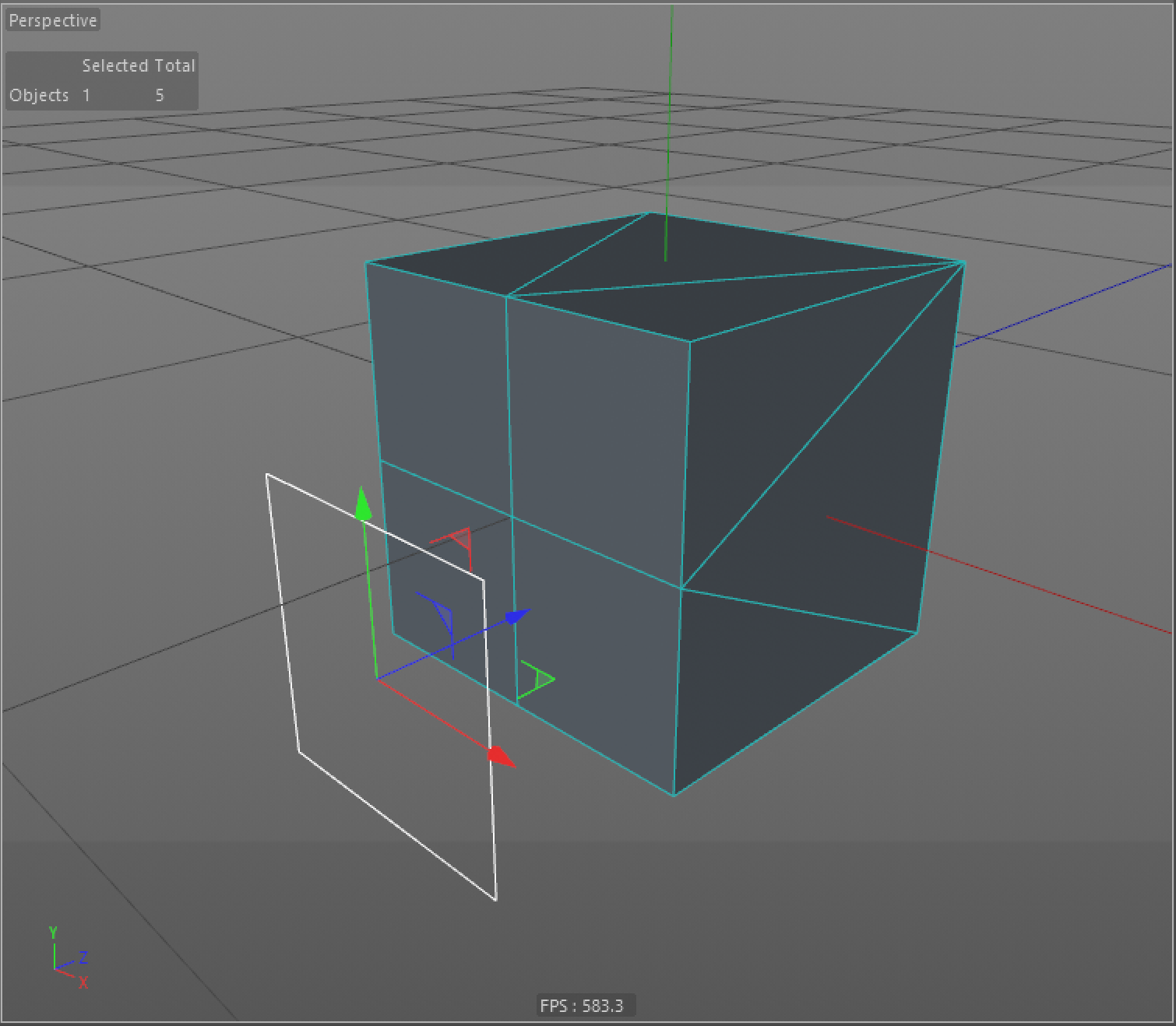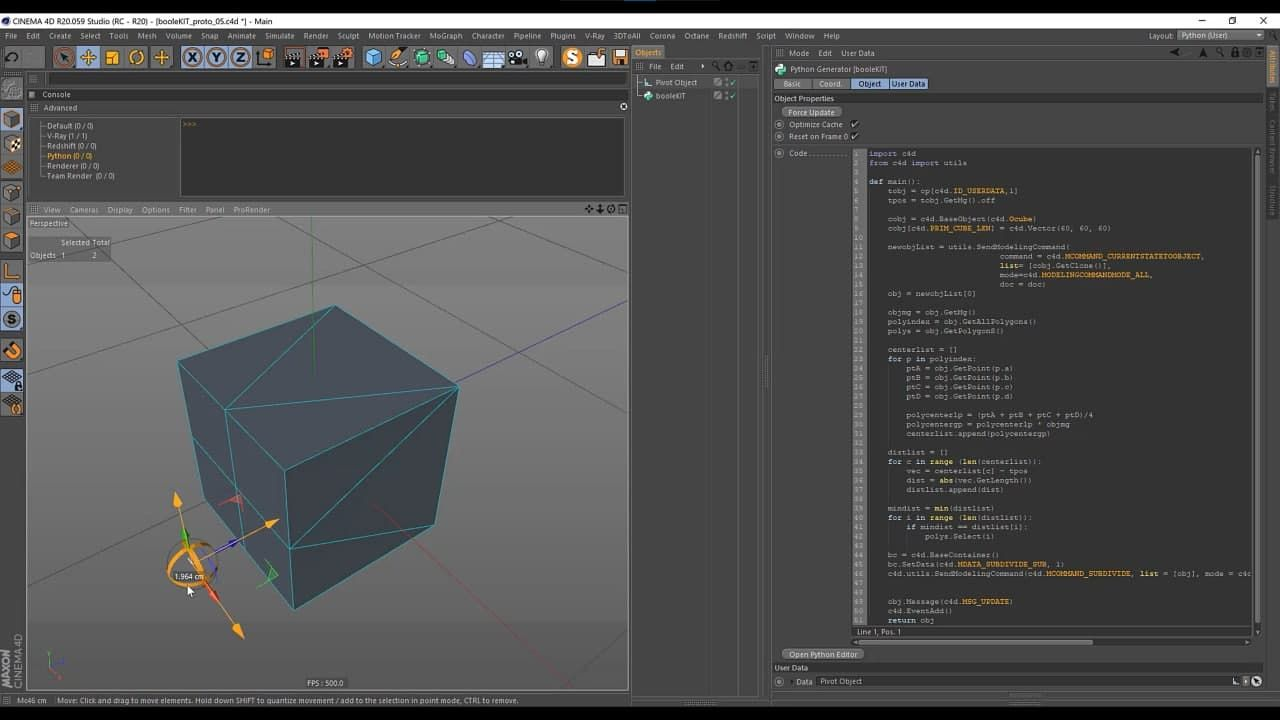# SOLVEDPG\ How to find polygon index

Hey SDK team,
I have a little problem with polygon index.

I'm looking for an Idea how to select the polygon (index) front in the target object (spl or poly).Does anybody have an idea how to find this index of polygon ?

Thanks a lot!

edit: In my head it sounds like this: i find the position of each polygon and compare with the position of the pointer object and then find the polygon index which is closest

edit 2: Do I need to use this post(https://developers.maxon.net/?p=194) in my case or is there another way?

note: I, @ferdinand, did consolidate your postings

Hey Ferdinand,
thank you for your answer. Yes, I'll be more accurate in my posts.
I found a solution like this, but I would like to clarify if it is optimal?``````def main():
tobj = op[c4d.ID_USERDATA,1]
tpos = tobj.GetMg().off

cobj = c4d.BaseObject(c4d.Ocube)
cobj[c4d.PRIM_CUBE_LEN] = c4d.Vector(60, 60, 60)

newobjList = utils.SendModelingCommand(
command = c4d.MCOMMAND_CURRENTSTATETOOBJECT,
list= [cobj.GetClone()],
mode=c4d.MODELINGCOMMANDMODE_ALL,
doc = doc)
obj = newobjList

objmg = obj.GetMg()
polyindex = obj.GetAllPolygons()
polys = obj.GetPolygonS()

centerlist = []
for p in polyindex:
ptA = obj.GetPoint(p.a)
ptB = obj.GetPoint(p.b)
ptC = obj.GetPoint(p.c)
ptD = obj.GetPoint(p.d)

polycenterlp = (ptA + ptB + ptC + ptD)/4
polycentergp = polycenterlp * objmg
centerlist.append(polycentergp)

distlist = []
for c in range (len(centerlist)):
vec = centerlist[c] - tpos
dist = abs(vec.GetLength())
distlist.append(dist)

mindist = min(distlist)
for i in range (len(distlist)):
if mindist == distlist[i]:
polys.Select(i)

bc = c4d.BaseContainer()
bc.SetData(c4d.MDATA_SUBDIVIDE_SUB, 1)
c4d.utils.SendModelingCommand(c4d.MCOMMAND_SUBDIVIDE, list = [obj], mode = c4d.MODELINGCOMMANDMODE_POLYGONSELECTION, bc=bc, doc = doc)

obj.Message(c4d.MSG_UPDATE)
return obj
``````

Hello @inevestenko,

That aside, a few points:

1. Computing the closest distance between two arbitrary geometries, e.g., a spline and a polygon object as you give as an example, can be quite complex.
2. Simplifying this to the distance between a point and an arbitrary polygon object will help you to understand this and must be implemented even if you want to do point one in the end.
3. You do not define what you consider to be the closest distance. For your example image you could ask for the shortest distance between the center of the plane gizmo and any of the center points of the polygons in the cube. But you could also ask for the two points contained in the plane gizmo and the cube object which form the shortest line-segment connecting these. And there are many other variants of this in between.

The Cinema 4D Python SDK does not offer you much which would help you here (the C++ SDK on the other hand has quite a few interfaces which would help). What you could use in Python is `c4d.utils.ViewportSelect`, although that would very much be an unintended usage. It is intended to carry out selections in a viewport. When you align the view transform, the camera, of a viewport with your plane gizmo, you could (try to) use it to retrieve the closest polygon. The method to retrieve the closest polygon, `ViewportSelect.GetNearestPolygon` does operate however with screen coordinates, as it is intended to handle mouse inputs, which makes this route unpractical.

The most simplistic interpretation of computing the distance would be to simply pick a query point p, then compute the polygon center points by taking the arithmetic mean of their vertices. The distance for each (p, center point) tuple is then the length of the vector difference of the two, e.g., `dist_3 = (center_point_polygon_3 - p).GetLength()`. To find the closest point in a polygon, i.e., not just pick the center point, you will have to do point plane projections. Assuming a mesh of triangles, project the query point onto plane defined by the triangle, using the dot product. Bring the projected point into barycentric coordinates and clamp them to the range [0, 1] which then will be the closest point q. Convert the barycentric coordinates back to cartesian and compute the distance between p and q. As before, do that for all polygons to find the closest one.

Helpful in this context might also be `c4d.utilsNiehgbor`. The more advanced acceleration data structures to make such computations fast, e.g., the Binary Space Partitioning stuff, are not exposed in the Python SDK. Finally, I must stress again, that your question is out of scope of support, which is why we cannot provide any further help here.

Cheers,
Ferdinand

Hey Ferdinand,
thank you for your answer. Yes, I'll be more accurate in my posts.
I found a solution like this, but I would like to clarify if it is optimal?``````def main():
tobj = op[c4d.ID_USERDATA,1]
tpos = tobj.GetMg().off

cobj = c4d.BaseObject(c4d.Ocube)
cobj[c4d.PRIM_CUBE_LEN] = c4d.Vector(60, 60, 60)

newobjList = utils.SendModelingCommand(
command = c4d.MCOMMAND_CURRENTSTATETOOBJECT,
list= [cobj.GetClone()],
mode=c4d.MODELINGCOMMANDMODE_ALL,
doc = doc)
obj = newobjList

objmg = obj.GetMg()
polyindex = obj.GetAllPolygons()
polys = obj.GetPolygonS()

centerlist = []
for p in polyindex:
ptA = obj.GetPoint(p.a)
ptB = obj.GetPoint(p.b)
ptC = obj.GetPoint(p.c)
ptD = obj.GetPoint(p.d)

polycenterlp = (ptA + ptB + ptC + ptD)/4
polycentergp = polycenterlp * objmg
centerlist.append(polycentergp)

distlist = []
for c in range (len(centerlist)):
vec = centerlist[c] - tpos
dist = abs(vec.GetLength())
distlist.append(dist)

mindist = min(distlist)
for i in range (len(distlist)):
if mindist == distlist[i]:
polys.Select(i)

bc = c4d.BaseContainer()
bc.SetData(c4d.MDATA_SUBDIVIDE_SUB, 1)
c4d.utils.SendModelingCommand(c4d.MCOMMAND_SUBDIVIDE, list = [obj], mode = c4d.MODELINGCOMMANDMODE_POLYGONSELECTION, bc=bc, doc = doc)

obj.Message(c4d.MSG_UPDATE)
return obj
``````

Hi @inevestenko,

yes, that looks like you are doing what I described under the first approach. But what jumps to the eye, is that you do not handle triangles as you always divide by four in `polycenterlp = (ptA + ptB + ptC + ptD)/4`. And although Cinema does store triangles as quads in the form of repeating the last vertex in a polygon, this will cause a problem for triangles.

When you have a `CPolygon` representing a triangle as a quad, i.e. A, B, C, C, then (A + B + C) * 1/3 != (A + B + C + C) * 1/4. So, you are probably calculating the wrong center point there. I might be overlooking something here, but that is what I get from a quick glance. But again, your question is out of scope of support, I am unfortunately not in the position to provide algorithmic support or debug your code for you.